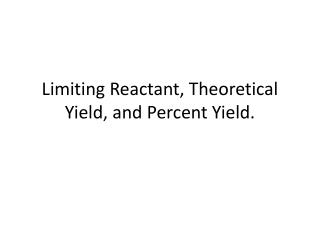# Limiting Reactant, Theoretical Yield, and Percent Yield. - PowerPoint PPT PresentationDownload PresentationLimiting Reactant, Theoretical Yield, and Percent Yield.

Limiting Reactant, Theoretical Yield, and Percent Yield.
Download Presentation## Limiting Reactant, Theoretical Yield, and Percent Yield.

- - - - - - - - - - - - - - - - - - - - - - - - - - - E N D - - - - - - - - - - - - - - - - - - - - - - - - - - -
##### Presentation Transcript

1. Limiting Reactant, Theoretical Yield, and Percent Yield.

2. A Problem to Consider… Which reactant is limiting if 20.0 g of iron (III) oxide (Fe2O3) are reacted with 8.00 g aluminum (Al) in the following thermite reaction? Fe2O3(s) + 2Al  2Fe(l) + Al2O3(s)

3. Fe2O3(s) + 2Al  2Fe(l) + Al2O3(s) • Step 1 Convert grams to moles • We have 20.0g of Iron(III) oxide, how many moles do we have? • We also have 8.00g of Aluminum, how many moles of aluminum do we have?

4. Fe2O3(s) + 2Al  2Fe(l) + Al2O3(s) • Step 2 Now determine how many moles of Fe can be produces from either reactant using the balanced chemical equation. • How many moles of Fe can Iron(III) oxide produce? • How many moles of Fe can the aluminum produce?

5. Limiting Reactant • There is enough Al to produce 0.297 mol Fe, but only enough Fe2O3 to produce 0.250 mol Fe. This means that the amount of Fe actually produced is limited by the Fe2O3 present, which is therefore the limiting reagent. • Fe2O3 is the Limiting Reactant

6. Theoretical Yield • You can only have as much of a product that the limiting reactant can provide so your theoretical yield is dictated by your limiting reactant. • In this scenario 20.0g Fe2O3 can only produce 0.250 moles of Fe, therefore 0.250 moles of Fe is the theoretical yield. • To determine the mass of 0.250 mol Fe, simply convert moles to grams.